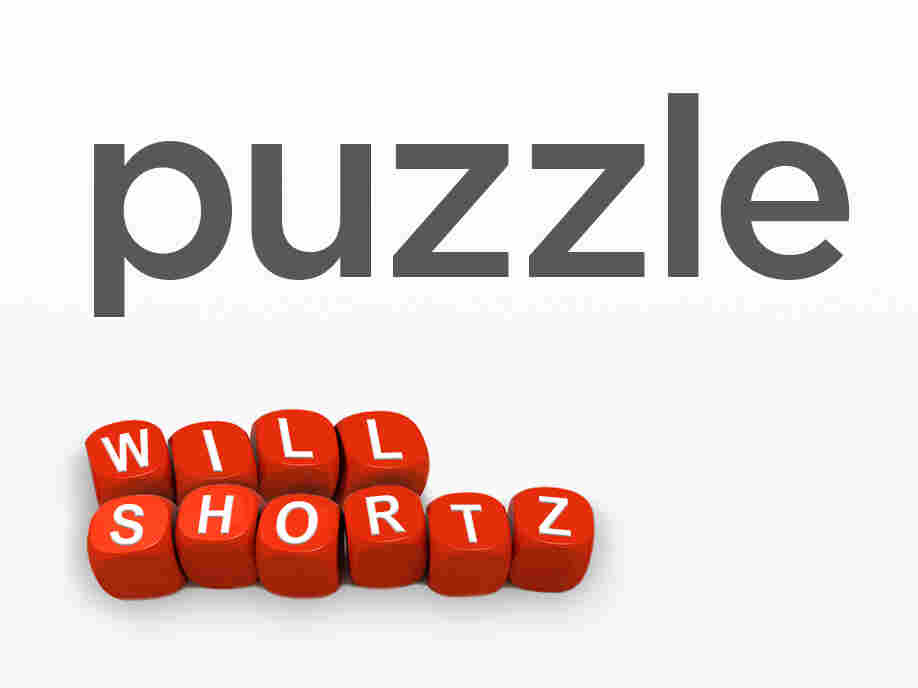Sunday Puzzle: Donate A Bit Of Your Time To This SuperPAC (Puzzle) This week's puzzle is called "SuperPACs." Every answer is a familiar two-word phrase or name in which the first word starts with PA- and the second word starts with C.#### Donate A Bit Of Your Time To This SuperPAC (Puzzle)

• `<iframe src="https://www.npr.org/player/embed/499893072/499952593" width="100%" height="290" frameborder="0" scrolling="no" title="NPR embedded audio player">`
• Transcript
Donate A Bit Of Your Time To This SuperPAC (Puzzle)

# Donate A Bit Of Your Time To This SuperPAC (Puzzle)

#### Donate A Bit Of Your Time To This SuperPAC (Puzzle)

• `<iframe src="https://www.npr.org/player/embed/499893072/499952593" width="100%" height="290" frameborder="0" scrolling="no" title="NPR embedded audio player">`
• TranscriptSunday Puzzle. NPR hide caption

toggle caption
NPR

Sunday Puzzle.

NPR

On-air challenge: This week's puzzle is called "SuperPACs." Every answer is a familiar two-word phrase or name in which the first word starts with PA- and the second word starts with C.

For example: Official who oversees a city's green spaces --> PARKS COMMISSIONER.

Last week's challenge: This is a two-week challenge. Take the digits 5, 4, 3, 2 and 1, in that order. Using those digits and the four arithmetic signs — plus, minus, times and divided by — you can get 1 with the sequence 5 - 4 + 3 - 2 - 1. You can get 2 with the sequence (5 - 4 + 3 - 2) x 1.

The question is ... how many numbers from 1 to 40 can you get using the digits 5, 4, 3, 2, and 1 in that order along with the four arithmetic signs?

You can group digits with parentheses, as in the example. There are no tricks to this, though. It's a straightforward puzzle. How many numbers from 1 to 40 can you get — and, specifically, what number or numbers can you not get?

Answer: 39 numbers — the only number you can not get is 39.

Here are the answers submitted by this week's winner. Note: Many numbers have multiple possible equations:

1=5-4+3-2-1;
2=(5-4+3-2)*1;
3=((5+4)/3) * (2-1);
4=(5+4-3-2)*1;
5=5*(4-3)*(2-1);
6=5-4+(3*2)-1;
7=5+4-3+2-1;
8=(5+4-3+2)*1;
9=5+4+3-2-1;
10=5+4+(3-2)*1;
11=5+4+3-2+1;
12=(5+4-3)*(2*1);
13=((5+4-3)*2)+1;
14=(5+4+3+2)*1;
15=5+4+3+2+1;
16=5+4+(3*2)+1;
17=5+(4*3)*(2-1);
18=5+(4*3)+2-1;
19=(5*4)-3+(2*1);
20=(5*4)-3+2+1;
21=(5*4)+3-(2*1);
22=(5*4)+3-2+1;
23=(5*4)+3*(2-1);
24=(5*4)+3+2-1;
25=(5*4)+3+(2*1);
26=(5*4)+3+2+1;
27=(5*4)+(3*2)+1;
28=5+(4*3*2)-1;
29=5+(4*3*2*1);
30=5+(4*3*2)+1;
31=((5*4*3)/2)+1;
32=5*(4+3)-2-1;
33=5*(4+3)-(2*1);
34=5*(4+3)-2+1;
35=5*(4+3)*(2-1);
36=5*(4+3)+2-1;
37=5*(4+3)+(2*1);
38=5*(4+3)+2+1;

(no equation for 39);

40=5*(4+3+2-1)

Winner: Margaret Gibbs of Littleton, Mass.

Next week's challenge, from listener Peter Gordon of Great Neck, N.Y.: Think of a name in the news that has a doubled letter. It's a person's last name. Change that doubled letter to a different doubled letter, and you'll get the commercial name for a popular food. What is it?